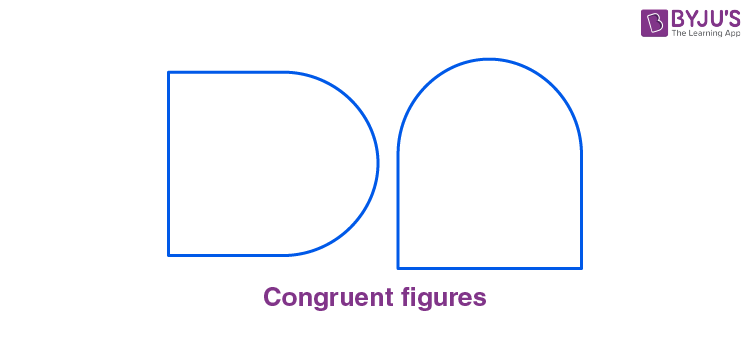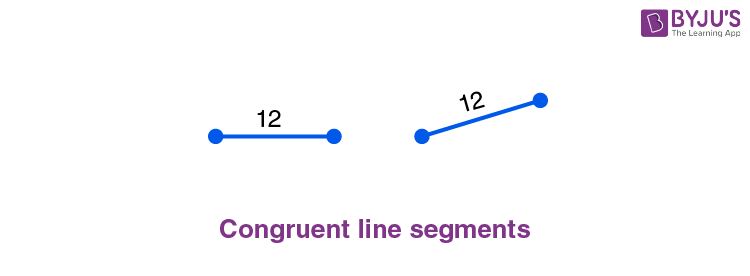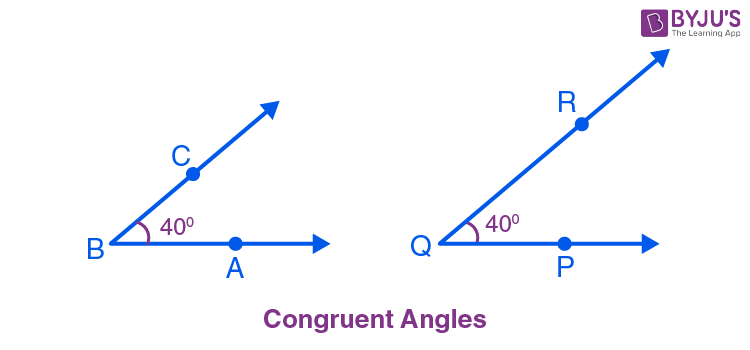# Congruence in Geometry - Congruent Figures and Lines

In Geometry, congruence is a term used to define two objects that have the same dimensions and shape. Moreover, if one shape can be exactly like the other on turning, flipping and/or sliding it, then the two shapes are said to be congruent to each other. So two congruent figures drawn on a piece of paper can be cut out and placed over one another to match up perfectly. When we say a figure A is congruent to a figure B, symbolically it can be written as figure A ≅ figure B. In this article, we will discuss the congruence of plane figures and line segments and congruent angles.

## Congruence of Plane Figures

You are given two plane figures. Using the method of superposition, here are the steps to find whether they are congruent to each other or not:• Trace a copy of figure 1 using tracing paper.
• Cut it out of the paper and place it over figure 2.
• You may slide or move or even turn over the paper. If they cover each other completely, they are congruent. We write figure 1 ≅ figure 2.

## Congruence Among Line Segments

Two line segments are said to be congruent to each other if they have equal lengths. They may or may not align at the same angle with an axis or position in the plane. To find whether one line segment is congruent to the other or not, we use the same method of superposition as discussed above. Thus if the two ends of the two-line segments lie on one another, they are congruent.## Congruent Angles

If two angles have the same measure in degrees, they are congruent angles. The angles may or may not lie in the same position or orientation on the plane. The method of superposition (as described above) can be used to check if given angles are congruent angles or not.Thus, ∠PQR ≅ ∠ABC because the two angles measure 40 degrees. It is to be noted here that the length of BC is not equal to the length of QR. But congruence of angles does not depend on the length of the arms of the angles; it depends on the measures of the angles only. Thus, ∠PQR and ∠ABC are congruent angles.